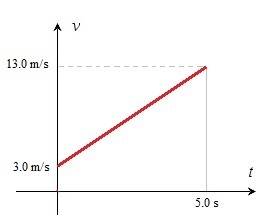# Acceleration – calculating the time

Up a level : Pre DP, Mechanics and Electronics
Previous page : Free fall - calculating the timeWe will continue with the rounded value of the acceleration of gravity on the Earth as a=g=10 m/s2.

We can find the height at which an thrown object is at by using a v/t-diagram, as we saw on the precious page, or by using the formula we found on the previous pages. To recapitulate, how far has a dropped object fallen after say 3 seconds?

If we choose up to be in the positive direction, then a v/t-diagram would  look something like this:The velocity after 3 s is  –10·3= –30 m/s, so the speed is 30 m/s. The area is now 3·30/2=45 m.  If we use the velocity we get -45 m. This because we choose up as the positive direction.

We could of course us the formula$s = \frac{{a{t^2}}}{2}$

This wound give us s=10·32/2=10·9/2=90/2=45 m as before.

Time from the displacement – starting at 0

Yet again, let us say we start at 0 m/s, and we ask how long time it would take to go 45 m? We start with the formula above and make the t the subject. This would give us$t = \sqrt {\frac{{2s}}{a}}$

Substituting in our values will give us$t = \sqrt {\frac{{2s}}{a}} = \sqrt {\frac{{2 \cdot 45}}{{10}}} = \sqrt {\frac{{90}}{{10}}} = \sqrt 9 = 3{\text{ s}}$

As expected we get the previous value back.

Time from displacement – starting at non zero

Let us say we have the situation described in the figure below.As we previously found out the displacement in the described movement is 5·(13+3)/2=5·16/2= 5·8=40 m.  The acceleration is (13–3)/5=10/5=2.0 m/s2.

Now let us say we know the starting velocity = 3.0 m/s, the acceleration = 2.0 m/s2, and that the displacement is 40 m, how to find the time?

We first solve this using the graph. As before we split it into a rectangle and a triangle.$s = 3t + \frac{{t \cdot 2t}}{2} = 3t + {t^2} = 40$

So how to solve this? If you are familiar with quadratic equations, you probably do now how to solve it. If not, then you could always resort to the surprisingly useful trial and error method.   In other words, test with some values. Let us start with say 1. This gives us 3·1+12=3+1=4 m. Too little. How abut 10? That would give us 3·10+102=30+100=140 m. Too much. How abut 5? That would give us 3·1+12=3+1=4 m. Too little. How abut 10? That would give us 3·5+52=15+25=40 m, just right.

Even if we would use more complicated values, say an initial velocity of 3.14 m/s and an acceleration of  say 5.12 m/s2 and thus have the equation$s = 3.14t + \frac{{t \cdot 5.12t}}{2} = 3.14t + 2.56{t^2} = 40$

it would be fairly easy to solve it by  testing. In the table below you can see my run.

 t  [s]: s [m]: 1 5.7 5 79.7 3 32.46 4 53.52 3.5 42.35 3.4 40.2696 3.37 39.655464 3.38 39.859664 3.39 40.064376

The value 3.39 s is close enough, and we have

40=3.14·3.39+2.56·3.392

I.e the displacement after 3.39 seconds is 40 metres.

The velocity would at that point be the 3.14 m/s we had to start with plus at=5.12·3.39=17.36 m/s. I.e. 20.5 m/s.

If you solve is using the quadratic formula or an equation solver on a calculator or computer  you will get t=3.39 s and t= –4.61 s. The first solution correspond to the one we are looking for.

Some deeper stuff that could be omitted in a first reading

So, does the negative time have any meaning? Let us “move back in time”.I think it is easiest to look at the motion starting at point of some obscure reason we have a stopwatch starting at t= –4.61 s.  At that point in time the object is at 40 meters from the object, in the positive direction,  and since the velocity is negative the object moves toward the origin and slowing down at a constant rate of 5.12 m/s2.

After a while the object are at the point B where the velocity is 0.  It will then start to gain velocity in the positive direction at a rate of 5.12 m/s2.

When the stopwatch shows the time 0 s the object has the velocity 3.14 m/s. Then it will reach the point C where the displacement yet again is 40 m.

The object will thus start at 40 m from the origin moving towards it, but slowing down until it reach the origin, then it will accelerate back again to reach the displacement 40 m again.

The yellow area correspond to a distance of 40 m, but so does the orange area minus the green.Up a level : Pre DP, Mechanics and Electronics
Previous page : Free fall - calculating the timeLast modified: Feb 9, 2017 @ 20:04